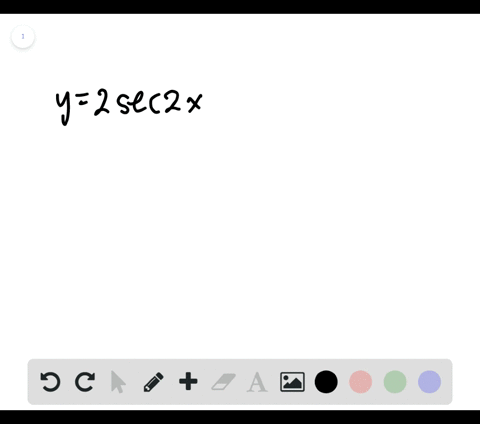🎉 The Study-to-Win Winning Ticket number has been announced! Go to your Tickets dashboard to see if you won! 🎉View Winning Ticket### Sketching the Graph of a Trigonometric Function I…

00:41University of Southern California
Problem 60

# Sketching the Graph of a Trigonometric Function In Exercises $55-66,$ sketch the graph of the function.$$y=\tan 2 x$$

## Discussion

You must be signed in to discuss.

## Video Transcript

problem Number 60 asks us to sketch the graph of the following function. Why equals tangent of two X Now, for any tangent function in the form y equals a tangent of B X. We know that the period of this function will always be equal to pi, divided by the absolute value. Now, in this case, B equals a positive too. So you don't need to add those absolute value science or they don't really change anything. But we find that our period is gonna be equal to Pi House instead of the standard period of pie for the tangent function. So what that means is I'm gonna come in here, mark up this number line, and I'm going to no tape High House Hi. Over here. Negative playhouse. So in red, at each interval of pie halfs, we're going to draw the asked meto declines that air characteristic of the tangent function exist here it zero as well. Hey, and then well, coming here and draw a tangent function. Remember the tangent exhibits asked Matilda behavior where as it approaches, each asked meto believer approaches positive infinity or negative infinity. And there you have it. That is how you would graph why equals tangent of two x